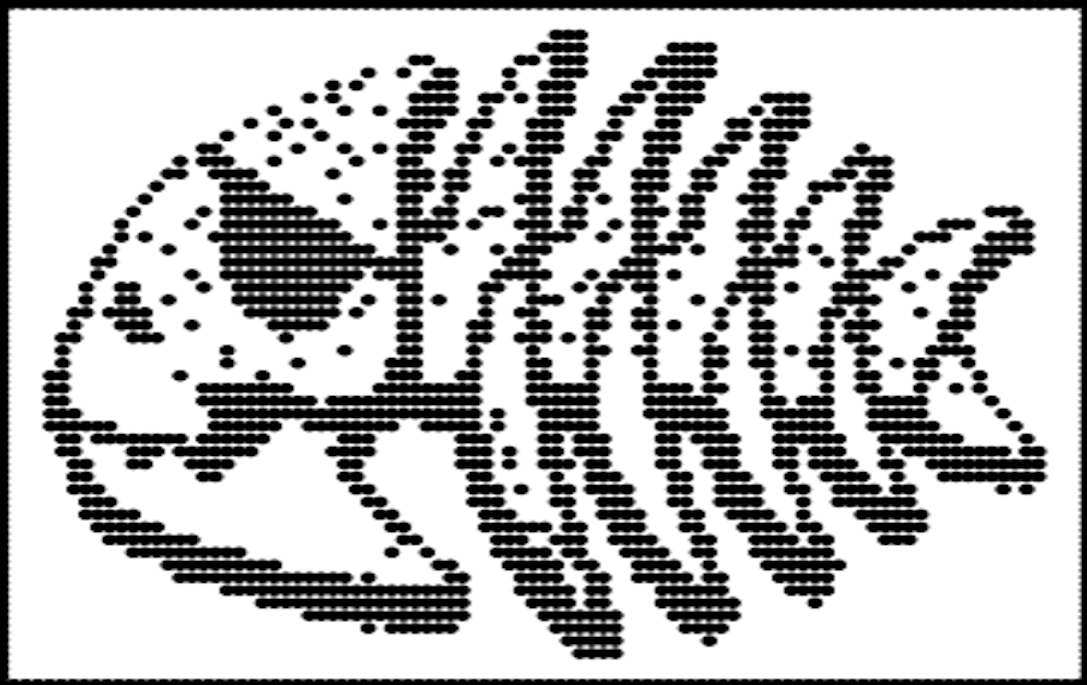1158 字

# 大隐隐于数

None of these assumptions was needed in deriving the optimal linear predictor.

convert 'fig.jpg'  -resize 100x100 -extent 100x100 -monochrome -compress none 'fig.pbm'

temp <- scan("~/logo.txt")

temp <- scan("fig.pbm", skip = 1)

save(data,file = 'data.RData')

load('data.RData')
reg <- lm(Y~X,data=data)
plot(reg$fitted,reg$resid,pch=16,main="Residual plot from data")